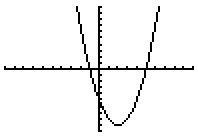When a quadratic function crosses the x-axis, those x-values are called the roots or zeros of the function. For a quadratic function that has real roots,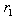and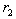, the factored form is given as: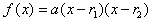; where a is a real number that works the same way as the a-value in the last lesson.
 For example: See how the graph of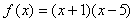intersects the x-axis at -1 and 5.